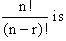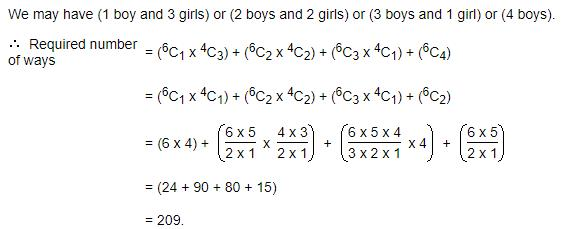Courses

# Test: Permutations Non Distinct Objects

## 20 Questions MCQ Test Mathematics (Maths) Class 11 | Test: Permutations Non Distinct Objects

Description
This mock test of Test: Permutations Non Distinct Objects for JEE helps you for every JEE entrance exam. This contains 20 Multiple Choice Questions for JEE Test: Permutations Non Distinct Objects (mcq) to study with solutions a complete question bank. The solved questions answers in this Test: Permutations Non Distinct Objects quiz give you a good mix of easy questions and tough questions. JEE students definitely take this Test: Permutations Non Distinct Objects exercise for a better result in the exam. You can find other Test: Permutations Non Distinct Objects extra questions, long questions & short questions for JEE on EduRev as well by searching above.
QUESTION: 1

### Sonia has 10 balloons out of which 5 are red, 2 white, 2 blue and 1pink, which she wants to use for the decoration. Her favourite pink colour balloon should be filled with toffees and should be put at the centre of the room above the cake table and remaining 9 at the wall behind the cake table. How many ways she can arrange the balloons?

Solution:

1 balloon is fixed that is to be placed above the cake
Now she have to arrange 9 balloons = 9!/(5!*2!*2!)
(9*8*7*6*5!)/((5!*2*2)
= (9*8*7*6)/(2*2)
= 756

QUESTION: 2

### In how many ways can 3 letters be posted in 4 letter boxes?

Solution:

The first letter can be posted in 4 ways. So, total outcomes about the first letter = 4.
For every outcome about the first letter, the second letter can be posted in 4 ways. So, total outcomes about the first and the second letters= (4*4) = 16.
Therefore, following the same route, we can say, total possible outcomes about the first and the second letters= (4*4*4) = 64.

QUESTION: 3

### Among 7 flags 4 are of red colour and the rest are all different colours. How mant different signals can be generated using these flags?

Solution:

Total flags = 4
Different colour = 3
red = 4
Total no. of signals = 7P3
= 7!/(4!)
= 210

QUESTION: 4

If (n + 1)! = 20(n – 1)!, then n is equal to

Solution:

(n + 1)! = 20 (n – 1)!
n (n + 1) = 20
(n – 4) (n + 5) = 0
Since, (n – 1)! exists, n ≥ 1
So, n = 4

QUESTION: 5

There are 3 white, 4 red and 1 blue marbles in a bag. They are drawn one by one and arranged in a row. Assuming that all 8 marbles are drawn, determine the number of different arrangements if marbles of same colour are indistinguishable.

Solution:
QUESTION: 6

The number of permutations of n different objects taken r at a time, where repetition is allowed

Solution:

Number of permutations of n different things taken r at a time when repetition is allowed = nr

QUESTION: 7

In a class 6 students have to be arranged for a photograph. If the prefect and the vice must occupy the positions at either ends, how many ways the students can be arranged?

Solution:

In a class of 6 students. Two students at both ends. Remaining four students have to be arranged
= 2 * 4!
= 2 * 24
= 48

QUESTION: 8

How many different words can be formed using the letters of the word BHARAT, which begin with B and end with T?

Solution:

If the first & last letter is fixed, then we find out, the number of permutations of the remaining letters, i.e. 4
= 4!/2!
= 4*3*2!/2!
= 12

QUESTION: 9

Find the number of words with or without meaning which can be made using all the letters of the word SWEET.

Solution:

Sweet has 5 letters, so it gives 5! but there are two letters which are same
Number of words will be = (5! ÷ 2!) = 60

QUESTION: 10

If n = 4 and r = 2, the value ofSolution:

n!/(n-r)!
4!/(4-2)!  = 4*3*2!/2!
= 12

QUESTION: 11

How many ways can three white and three red balloons be arranged in a row?

Solution:

3 white balls, 3 red balls
Total balls = 6
Total no. of ways = (6!)/(3!)(3!)
= (6*5*4*3!)/(3!*3*2)
= (5*4) = 20

QUESTION: 12

Out of 7 consonants and 4 vowels, how many words of 3 consonants and 2 vowels can be formed?

Solution:

1/4! + 1/5!
= 1/4![1 + 1/5]
1/4![6/5]
=> 6/5!

QUESTION: 13

In a room there are 2 green chairs, 3 yellow chairs and 4 blue chairs. In how many ways can Raj choose 3 chairs so that at least one yellow chair is included?

Solution:

At least one yellow chair means “total way no yellow chair
Total ways to select 3 chairs form the total (2+3+4) chairs 9C3
9C3 = 9!/3!*6! = 84ways
Now, we dont want even one yellow chair
So, we should select 3 chairs from 6 chairs (2green & 4blue) = 6C3
6C3 = 6!/3!3! = 20 ways
hence , ways to select at least one yellow chair = 84-20 = 64 ways.

QUESTION: 14

In how many ways can 10 people line up at a ticket window of a cinema hall?

Solution:

If you imagine there are 10 spots on the floor in a line where people can stand, then the first person has 10 choices of where to stand.
The second person has 9 choices since the first person is already on one of the spots.
The third person has 8 choices. And so on until the last person has no choice.
We can now mulitply the numbers together. 10x9x8x7x6x5x4x3x2x1. This is called a factorial (where you multiply all of the consecutive numbers together from 1) and it is written as 10!.
This means there are 3,628,800 ways of arranging the 10 people

QUESTION: 15

The number of permutation of n objects, where p1 objects are of one kind, pobjects are of second kind, and so on till pk objects are of kth kind and rest, if any, are of different kinds is

Solution:

By theorem, The number of permutations of n objects, where p1 objects are of one kind, p2 are of second kind, ..., pk are of kth kind and the rest, if any, are of different kind is n!/(p1! * p2! *......* pk!)

QUESTION: 16

In a group of 6 boys and 4 girls, four children are to be selected. In how many different ways can they be selected such that at least one boy should be there?

Solution:QUESTION: 17

Number of signals that can be made using given 4 flags of which 3 are blue and 1 is red?

Solution:

Total flags = 4
Blue = 3
red = 1
Total no. of signals = (4!*3!)/3!
= 4

QUESTION: 18

A coin is tossed 6 times, in how many throws can 4 heads and 2 tails be obtained?

Solution:

To begin with, we calculate the total number of possibilities that arise from tossing a coin 6 times . On each toss , we have 2 possibilities - a head or a tail. This gives us
2*2*2*2*2*2 = 64 possibilities
Now let's list out the desirable outcomes.
H H H H T T - This is one example of the above outcome. Something like
H H H T H T would also be equally likely and would be a desirable outcome. Thus to calculate all such permutations
= (6!/4!) ∗ 2!
= 15ways

QUESTION: 19

Which of the following options is true

Solution:
QUESTION: 20

How many three letter codes can be formed by only vowels of English alphabets, given that repetition of letters is allowed?

Solution:

We have 5 choices (a, e, i, o, u)
3 letter codes = 5 * 5 * 5
= 125